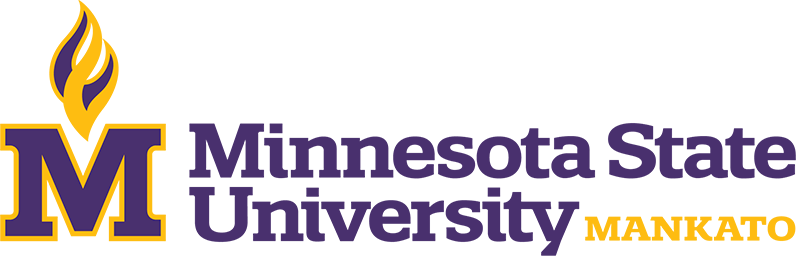The Department of Mathematics and Statistics offers four master’s programs: Mathematics MA, Mathematics and Statistics MS, Applied Statistics MS, and Mathematics Education MS. The Mathematics MA has three emphases: a broad selection of courses in mathematics, mathematics and computer sciences, and mathematics and community college teaching.

The department consists of approximately twenty-four faculty members, including most faculty members with a Ph.D. in mathematics, statistics, or mathematics education. The department supports approximately 30 graduate students per year. Faculty specialties or interests include abstract algebra, applied computational mathematics, combinatorial matrix theory, complex analysis, discrete mathematics and combinatorics, dynamical systems, elementary and secondary mathematics education, differential geometry, symplectic geometry, industrial mathematics, mathematical biology, numerical analysis, optimization, partial differential equations, functional analysis, real analysis, applied statistics, Bayesian analysis, mathematical statistics, time series and survival analysis, stochastic processes, actuarial science, topology, big data, and data analytics.

Graduates of the program have found employment in a variety of fields, including software engineering, graphic design, insurance, community college teaching, secondary mathematics teaching and as statisticians or data scientists. Others have gone on to obtain a Ph.D. in mathematics or statistics.